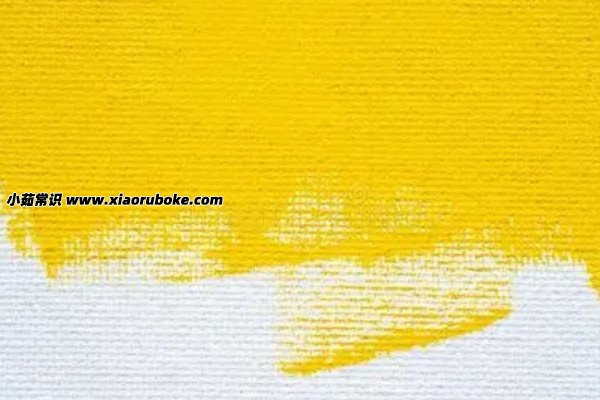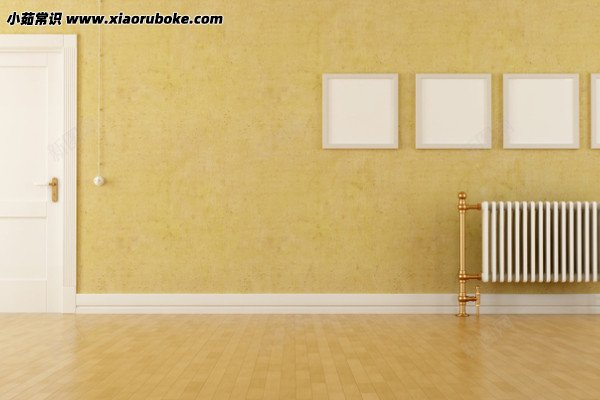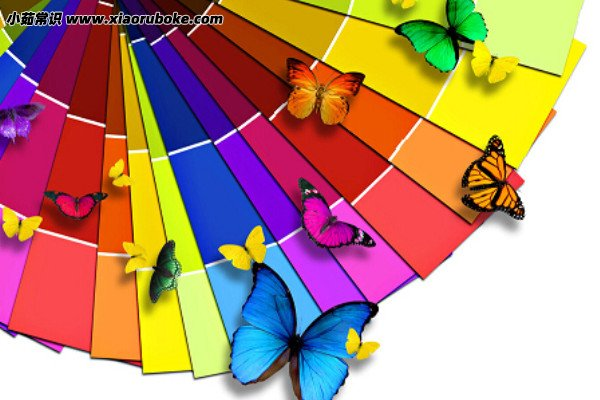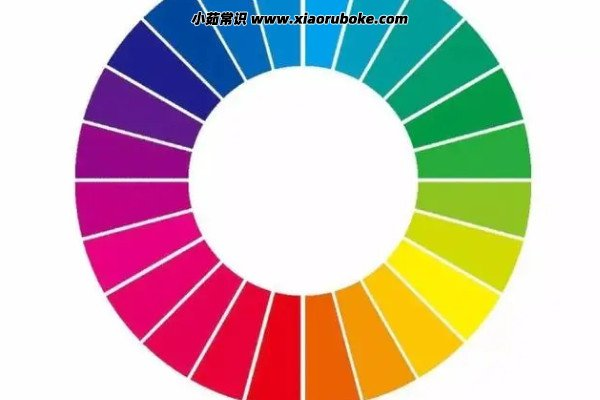﻿﻿ 黄色加白色是什么颜色，相同比例混合为米黄色(颜色和配比有关) — 小茹常识

# 黄色加白色是什么颜色，相同比例混合为米黄色(颜色和配比有关)

## 黄色加白色是什么颜色## 颜色调色搭配大全1. 暗红=玫红色+黑色(少量)

2. 禇石红=白色+红色+黑色(少量)

3. 玫瑰红+柠檬黄+纯黑色

4. 粉玫红=玫红色+白色

5. 玫瑰红=大红+紫罗兰

6. 中黄=大红+桔黄

7. 米黄色=黄色+白色

8. 藤黄色=柠檬黄+玫瑰红

9. 桔黄色=柠檬黄+玫瑰红

10. 粉蓝=蓝色+白色

11. 墨蓝=天蓝色+黑色(少量)

12. 粉蓝色=纯白色+天蓝色

13. 灰蓝色=天蓝色+纯黑色

14. 浅灰蓝=天蓝色+纯黑色+纯紫色

15. 浅灰蓝=天蓝色+黑色

16. 浅绿=柠檬黄+草绿

17. 草绿=柠檬黄+湖蓝

18. 嫩绿=天蓝色+黄色

19. 蓝绿=天蓝色+草绿色

20. 墨绿=草绿色+少量黑色21. 粉绿色=纯白色+草绿色

22. 青莲=湖蓝+紫罗兰

23. 粉紫色=纯白色+纯紫色

24. 浅蓝紫=天蓝色+黑色+紫

25. 紫罗兰=大红+湖蓝

26. 熟褐色=柠檬黄+纯黑色+玫瑰红

27. 啡色朱=红色+黑色少量

28. 啡色=玫瑰红+纯黑色

29. 香蕉灰=柠+黄绿+白

30. 暖梨灰=土黄+橄

31. 冷固灰=土黄+黄绿

32. 冷重灰=黑(稀释)+普兰

33. 暖重灰=黑(稀释)+深红

34. 瓦蓝灰=普蓝+粉绿+紫罗兰(少量)

35. 太平洋灰=青莲+天蓝+普兰+粉绿

36. 黄绿色=柠檬黄+草绿色

37. 墨绿色=草绿色+纯黑色

38. 草绿、嫩绿=天蓝色+黄色

39. 粉柠檬黄=柠檬黄+纯白色

40. 人物的皮肤颜色=红色+黄+白